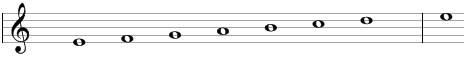# Phrygian scale

The Phrygian scale is a mode of the primary heptatonic scale that can be built using the following steps between notes: ½, 1, 1, 1, ½, 1, 1.

One example of the scale is E, F, G, A, B, C, D. This example is shown below in traditional notation and in guitar tablature notation.The Phrygian scale is the third mode of standard major scale or the fifth mode of the natural minor scale.

The Phrygian scale is a "primary" heptatonic scale (heptonia prima) as the intervals between any two adjacent notes are either one or two semitones (1 step or ½ steps) and as the two single-semitone intervals are spaced as evenly as possible (going up from the single-semitone interval between E and F we will find three two-semitone intervals - between F and G, G and A, and A and B – before we get to the second single semitone interval between B and C; going in the opposite direction from the single-semitone interval between F and E we will encounter two two-semitone intervals – between E and D and D and C – before we reach again the single-semitone interval between B and C).

## Modes of the Phrygian scale

The following are modes of the Phrygian scale.

1. Phrygian, with steps ½, 1, 1, 1, ½, 1, 1 (E, F, G, A, B, C, D in the example above).
2. Lydian, with steps 1, 1, 1, ½, 1, 1, ½ (F, G, A, B, C, D, E).
3. Mixolydian, with steps 1, 1, ½, 1, 1, ½, 1 (G, A, B, C, D, E, F).
4. Aeolian, also known as the natural minor or the melodic descending minor, with steps 1, ½, 1, 1, ½, 1, 1 (A, B, C, D, E, F, G).
5. Locrian, with steps ½, 1, 1, ½, 1, 1, 1 (B, C, D, E, F, G, A).
6. Ionian or major, with steps 1, 1, ½, 1, 1, 1, ½ (C, D, E, F, G, A, B).
7. Dorian, with steps 1, ½, 1, 1, 1, ½, 1 (D, E, F, G, A, B, C).

## Three-note chords on the Phrygian scale

The following are common triads built on the notes of the Phrygian scale.

• On the root of the scale (on the tonic): minor chord (e.g., Em composed of E, G, and B) or suspended chord (Esus4 = E, A, B).
• On the second note (on the supertonic): major chord (F = F, A, C) or suspended chord (Fsus2 = F, G, C).
• On the third note (on the mediant): major chord (G = G, B, D) or suspended chords (Gsus4 = G, C, D and Gsus2 = G, A, D).
• On the fourth note (on the subdominant): minor chord (Am = A, C, E) or suspended chords (Asus4 = A, D, E and Asus2 = A, B, E).
• On the fifth note (on the dominant): diminished chord (Bdim = B, D, F).
• On the sixth note (on the submediant): major chord (C = C, E, G) or suspended chords (Csus4 = C, F, G and Csus2 = C, D, G).
• On the seventh note (on the leading tone): minor chord (Dm = D, F, A) or suspended chords (Dsus4 = D, G, A and Dsus2 = D, E, A).

## Four-note chords on the Phrygian scale

The following are seventh chords built on the notes of the Phrygian scale.

• On the first note: minor seventh chord (e.g., Emin7 composed of E, G, B, and D).
• On the second note: major seventh chord (Fmaj7 = F, A, C, E).
• On the third note: dominant seventh chord (G7 = G, B, D, F).
• On the fourth note: minor seventh chord (Amin7 = A, C, E, G).
• On the fifth note: half-diminished seventh chord (minor seventh chord with a flat fifth, Bm7b5 = B, D, F, A).
• On the sixth note: major seventh chord (Cmaj7 = C, E, G, B).
• On the seventh note: minor seventh chord (Dmin7 = D, F, A, C).

## Intervals on the Phrygian scale

The Phrygian scale is composed of the following intervals.

• A minor second, e.g., the interval between E and F is equal to one semitone.
• A minor third, e.g., the interval between E and G is equal to three semitones.
• A perfect fourth, e.g., the interval between E and A is equal to five semitones.
• A perfect fifth, e.g., the interval between E and B is equal to seven semitones.
• A minor sixth, e.g., the interval between E and C is equal to eight semitones.
• A minor seventh, e.g., the interval between E and D is equal to ten semitones.

Scale, Scale (index)

### Filtered HTML

• Freelinking helps you easily create HTML links. Links take the form of [[indicator:target|Title]]. By default (no indicator): Click to view a local node.